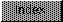```

NAME
IEEEDPCeil -- compute Ceil function of IEEE double precision number

SYNOPSIS
x   = IEEEDPCeil(  y  );
d0/d1              d0/d1

double  x,y;

FUNCTION
Calculate the least integer greater than or equal to x and return it.
This value may have more than 32 bits of significance.
This identity is true.  Ceil(x) = -Floor(-x).

INPUTS
y -- IEEE double precision floating point value

RESULT
x -- IEEE double precision floating point value

BUGS